# Generate Logistic Map in Fortran

I had been following the MOOC “Scientific Computing with Fortran” for a week and currently doing the exercises. This is one of the assignments which has piqued my interests - Logistic map. Here’s a neat animation from Wikimedia for the same.As Wikipedia states it, “The logistic map is a polynomial mapping (equivalently, recurrence relation) of degree 2, often cited as an archetypal example of how complex, chaotic behaviour can arise from very simple non-linear dynamical equations”. It is given by the equation, $$x_{n+1} = rx_n(1-x_n)$$ where,

• $x_n \in (0, 1)$
• $r \in [-2,4]$ (just to keep the final $x_n$ values bound to $[-0.5, 1.5]$)

I have written the following Fortran code (along with a simple GnuPlot script) to generate the plot.

  1 2 3 4 5 6 7 8 9 10 11 12 13 14 15 16 17 18 19 20 21 22 23 24 25 26 27 28 29 30 31 32 33 34 35 36 37 38 39 40 41 42 43 44 45 46 47 48 49 50 51 52 53 54 55 56 57 58 59 60 61 62 63 64 65 66 67  program logisticmap2 use, intrinsic :: iso_fortran_env, only : error_unit, dp=>real64 ! i, j, k are integers used for indexing implicit integer(i-k) ! n is the number of iterations ! r_n is the number of elements in r (ranging from r0 to 4) ! x0_n is the number of elements in initial x value, ranging from 0 to 0.99 integer, parameter :: n=3, r_n=1001, x0_n = 101 real(dp) :: r_vals(r_n), x0_vals(x0_n), xn, r0 ! xn_vals is a (r_n x x0_n) matrix for final output real(dp) :: xn_vals(r_n, x0_n) ! Parameters for IO integer :: istat, fn character(len=1024) :: msg ! Getting the initial r value from user print *, "Enter initial r0 value: " read(*,*) r0 ! Generate r and x0 values r_vals=linspace(r0, 4._dp, r_n) x0_vals=linspace(0.0_dp, 0.99_dp, x0_n) ! Generate and fill data do i = 1,x0_n xn = x0_vals(i) do j = 1, r_n do k= 1, n xn = r_vals(j)*xn*(1-xn) end do xn_vals(j, i) = xn end do end do ! Write to txt file open(newunit=fn, file='logisticmap2.txt', access='sequential', & status='old', action='write', form='formatted', iostat=istat, iomsg=msg) ! Simpler command is as follows: ! open(newunit=fn, file='logisticmap2.txt') do i=1, r_n write(fn, *) r_vals(i), xn_vals(i,:) if (istat /= 0) then ! Error handling, in case write(error_unit, *) msg close(fn) stop 1 end if end do close(fn) ! calling the gnuplot to plot logisticmap2.plt file call execute_command_line('gnuplot -p logisticmap2.plt') contains ! A linspace function similar to matlab function linspace(x0, xn, n) result(x) integer, intent(in):: n integer :: i real(dp), intent(in):: x0, xn real(dp) :: x(n) real :: step step=(xn-x0)/(n-1) do i=1,n x(i) = x0 + step*(i-1) end do end function linspace end program logisticmap2 

And the following is store in the logisticmap2.plt file.

 1 2 3 4 5 6 7  set nokey set grid set title "Logistic Map X_{n+1}=r X_n(1-X_n) with (n=3)" set xlabel "r" set ylabel "X_n" m="logisticmap2.txt" plot for [col=2:101] m using 1:col with d 

I have generated the plot for various initial r values,which pertains to different zoom levels.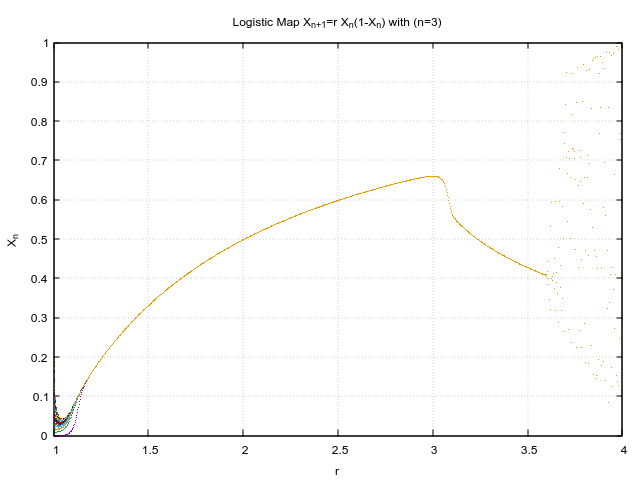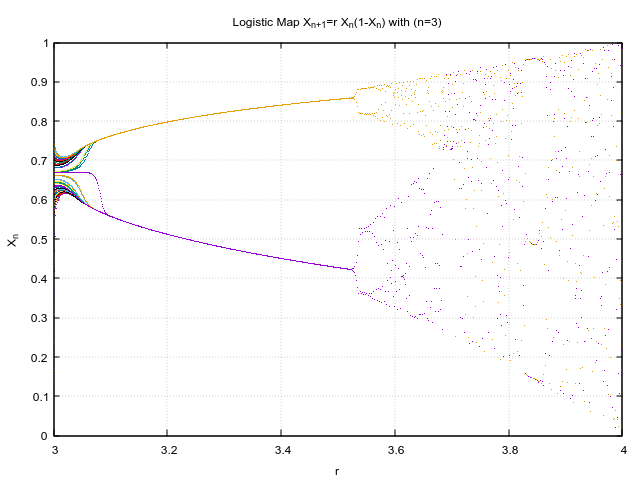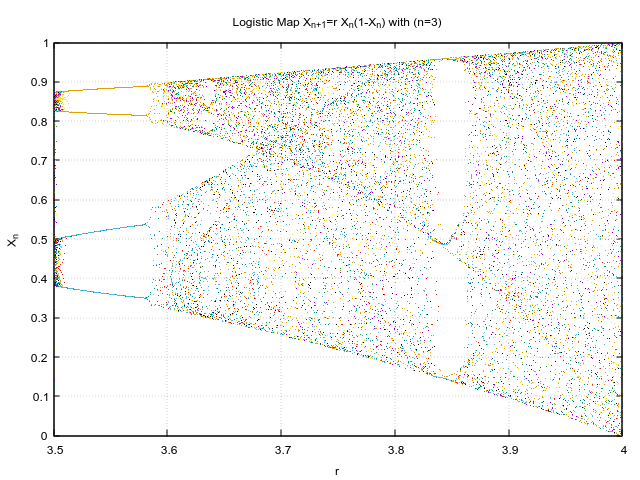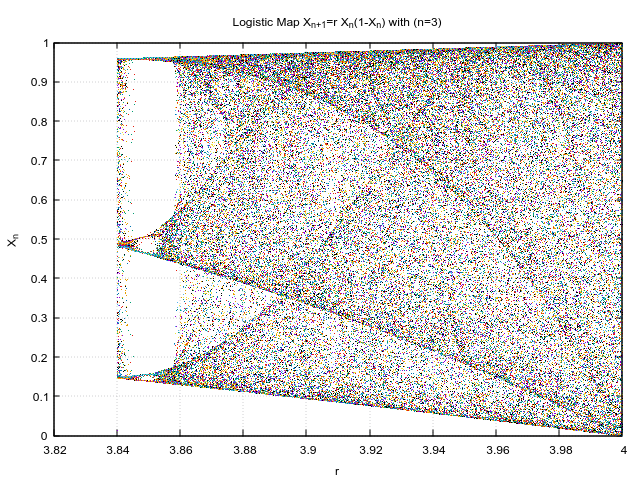I like how such chaotic behaviour rises out of seemingly simple equation and that too in just 3 iterations! Just to put in perspective, this is how the initial conditions looked like.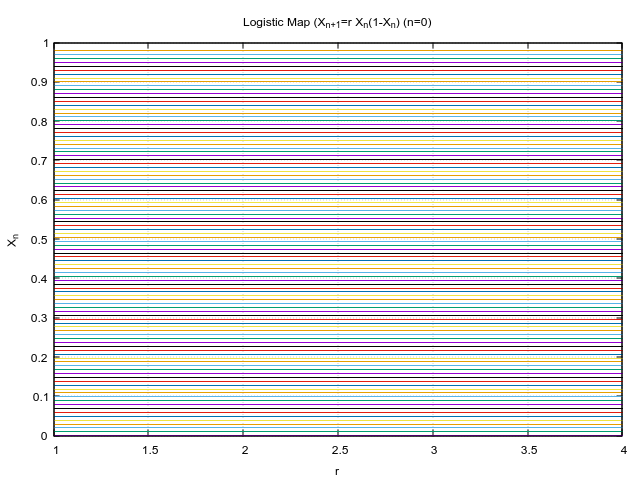:)